Courses

# Questions:Linear Algebra and Matrices - Mathematical Methods of Physics, UGC - NET Physics Physics Notes | EduRev

## Physics: Questions:Linear Algebra and Matrices - Mathematical Methods of Physics, UGC - NET Physics Physics Notes | EduRev

The document Questions:Linear Algebra and Matrices - Mathematical Methods of Physics, UGC - NET Physics Physics Notes | EduRev is a part of the Physics Course Physics for IIT JAM, UGC - NET, CSIR NET.
All you need of Physics at this link: Physics

Question 1. Let Mn be the vector space of all n × n matrices with real entries under usual matrix addition and scalar multiplication. Find the dimensions of the fol lowing subspaces of Mn:

1. W1 = {A ∈ Mn : A is symmetric}
2. W2 = {A ∈ Mn : A is skew-symmetric}
3. W3 = {A ∈ Mn : A is upper triangular}
4. W4 = {A ∈ M: A is tridiagonal}

Solution. It is to be noted that the dimensions of the above subspaces can be found just by calculating the number of entries which we can choose freely to construct a matrix of the given type. For symmetric matrices, aij = aji , so that we are not free to choose the (j, i)th entry once we choose the (i, j )th entry i.e. once we choose the (i, j )th entry, the (j, i)th entry is automatically chosen. Thus, the dimension of W1 is just the number of entries on and above the diagonal of an n × n matrix. So, dim(W1) = n + (n − 1) + (n − 2) + . . . + 2 + 1 =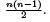For skew-symmetric matrices, aij = −aji and so the diagonal entries are all zero. Also, as above, the selection of (i, j )th entry ﬁnalizes the (j, i)th entry too. So, the dimension of Wis just the number of entries above the diagonal of an n × n matrix. Hence, dim(W1) = (n − 1) + (n − 2) + . . . + 2 + 1 =Similarly, dim (W3 ) =Now, for a tridiagonal matrix, the entries in all other positions except in the main diagonal, the ﬁrst diagonal above it and the ﬁrst diagonal below it are always zero. So the dimension of W4 is just the number of entries in these three diagonals of an n × n tridiagonal matrix. There are two entries each in the ﬁrst and the last rows and three each in all other rows. So, dim(W4 ) = 2 + 3(n − 2) + 2 = 3n − 2.

Question 2. Let V be the subspace of M2(R) consisting of matrices such that the entries of the ﬁrst row add up to zero. Write down a basis for V.
Solution.
The matrices in V will be of the form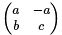Hence, one possible basis is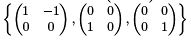Question 3. Write down a necessary and suﬃcient condition, in terms of a, b, c and d (which are assumed to be real numbers), for the matrix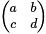not to have a real eigenvalue.

Solution. Clearly, this matrix won’t have any real eigenvalues if and only if its characteristic polynomial has all imaginary zeros.
Thus, (a + d)2 < 4(ad − bc).

Question 4. Given that the matrix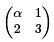has 1 as an eigenvalue, compute its trace and its determinant.
Solution. Let the other eigenvalue be x. Then α + 3 = 1 + x and 3α − 2 = x, so that α = 2. so, trace=5 and determinant=4

Question 5. Let A be a non diagonal 2 × 2 matrix with complex entries such that A = A−1. Write down its characteristic and minimal polynomials.
Solution. Since A = A−1, so det(A) = ±1. But, for A to be non-diagonal matrix, det(A) = −1. Thus, A is of the form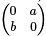where ab = 1. Since A2 = I , so the characteristic polynomial of A is x− 1 and the minimal polynomial is also x2 − 1.

Question 6. For a ﬁxed positive integer n ≥ 3, let A be the n × n matrix deﬁned by A = I −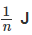, where J is the n × n matrix with all entries equal to 1. Which of the fol lowing statements is not true?

1. Ak = A for every positive integer k.
2. Trace(A) = n − 1
3. Rank(A) + Rank(I − A) = n
4. A is invertible.

Solution. Simple matrix multiplication reveals that A2 = A and hence it can be shown that Ak = A for every positive integer k, so that (1) is true.
The simplest thing that we can check is

Trace(A) = Trace(I ) − T race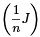= n − 1

Hence (2) is true.
Now, since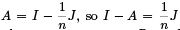and so rank(I − A) = 1. Also, performing the elementary operations Rn → Rn + Rn − 1 + . . . + R1 and then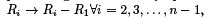it is clear that Rank(A) = n − 1 and so (3) is true.

Now, it can be seen that x = (1, 1, . . . , 1)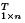satisﬁes Ax = 0, so that 0 is an eigenvalue of A. Hence A is singular and so A is not invertible. So, (4) is not true.

Question 7. Let A be a 5 × 4 matrix with real entries such that Ax = 0 if and only if x = 0, where x is a 4 × 1 vector. Then ﬁnd the rank of A.
Solution.
From the question it is clear that the homogeneous system of equations Ax = 0 has only trivial solution. So, Rank(A) = the number of unknowns = 4.

Question 8. Consider the following row vectors

a1 = (1, 1, 0, 1, 0, 0), a2 = (1, 1, 0, 0, 1, 0),
a3 = (1, 1, 0, 0, 0, 1) a= (1, 0, 1, 1, 0, 0),
a5 = (1, 0, 1, 0, 1, 0), a6 = (1, 0, 1, 0, 0, 1)

What is the dimension of the real vector space spanned by these vectors?
Solution
. Simple elementary operations will reveal that only four of these vectors are linearly independent. So, dimension of the vector space spanned by these vectors is 4.

Question 9. Let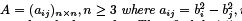i, j = 1, 2, . . . , n for some distinct real numbers b1 , b2 , . . . , b. Then ﬁnd det(A).

Solution. Performing the elementary operations, Ri → Ri − R1 for each i = 2, 3, . . . , n we see that det(A) = 0. Note that det(A) may not be zero if n = 2.

Question 10. Number of matrices with real entries, of the form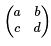such that A− A = 0 is

1. only one
2. only two
3. ﬁnitely many
4. inﬁnitely many

Solution. Using the equation A2 = A, it can be shown that any matrix A of the form A =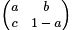where bc = a − a2 , satisﬁes the given condition. So, there are inﬁnitely many such matrices.

Question 11. Let V be a vector space of dimension n over the ﬁeld Zp where p is a prime. Then how many elements are there in V ?
Solution.
Since dim(V ) = n, so let {e1 , e, . . . , en } be a basis of V . Then any element of V is a linear combination of the form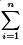αi ewhere αi ∈ Z. So,
the number of elements in V is pn .

Question 12. If T :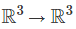be a linear transformation deﬁned by T (1, 0, 0) = (1, 0, 1), T (0, 1, 0) = (0, 0, 1) and T (0, 0, 1) = (1, 0, 0). Then ﬁnd the range space of T , null space of T , rank and nullity of T

Solution. Clearly, T (x, y, z ) = xT (1, 0, 0)+yT (0, 1, 0)+zT (0, 0, 1) = (x+z , 0, x+ y) = (x + z )(1, 0, 0) + (x + y)(0, 0, 1). Thus the range space of T is {(x, 0, y) : x, y ∈ R}. Also, T (x, y, z ) = 0 ⇒ x = −y = −z and so the null space of T is {(x, −x, −x) : x ∈ R}. Rank(T )= Dimension of the range space=2 and nullity(T )=Dimension of the null space of T =1.

Question 13. Let A ∈ M2(R) such that tr(A) = 2 and det(A) = 3. Write down the characteristic polynomial of A−1 .

Solution. Here, the characteristic polynomial of A is x2 −(sum of eigenvalues)x+ (product of eigenvalues) i.e x2 − tr(A)x+ det(A). By Cayley Hamilton Theorem,

A2 − tr(A)A + det(A)I = 0

⇒A2 − 2A + 3I = 0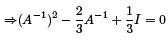Thus the characteristic polynomial of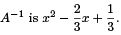Question 14. Let a 3 × 3 matrix A have eigenvalues 1, 2, −1, then ﬁnd the trace of the matrix B = A − A−1 + A2 .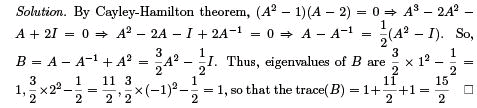Question 15. The eigenvectors of a 3 × 3 matrix A corresponding to the eigenvalues 1, 1, 2 are (1, 0, −1)T , (0, 1, −1)T and (1, 1, 0)T . Find the matrix A.
Solution.
Let A =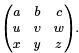Then, we can compute the unknowns using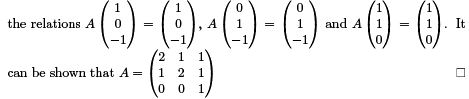Question 16. What is the null space of the matrix A =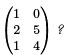Solution. Its easy to see that the rank of A is 2 and so by rank-nullity theorem, the nullity of A is 0, so that the null space of A is the zero space.

Question 17. Let V be the vector of all n × n matrices over the ﬁeld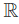of real numbers. A subset W of V is not a subspace of V if W consists of all matrices for which

1. A2 = A
2. A2 ≠ A
3. A = AT
4. A = −AT

Solution. Clearly, under the restriction A2 ≠ A, the zero vector cannot belong to W and so W cannot be a subspace of V .

Question 18. Let A be a 4 × 4 invertible matrix. Which of the following is NOT true?

1. The rows of A form a basis of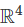2. Null space of A contains only the zero vector
3. A has four distinct eigenvalues
4. Image of the linear transformation x → Ax on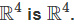Solution. Since A is invertible, so rank(A) = 4 and so the rows of A are linearly independent and hence they form a basis of. Since nullity(A) = 0, so the null space of A contains only the zero vector. It can be seen that for e1 = (1, 0, 0, 0)T , e2 = (0, 1, 0, 0)T , e3 = (0, 0, 1, 0)T , e4 = (0, 0, 0, 1)T , their images Ae1, Ae2, Ae3 and Ae4 are the columns of A, which are linearly independent as rank(A) = 4. But it is not necessary that A has distinct eigenvalues. Thus option 3 is NOT correct.

Question 19. Let C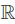be the linear space of all continuous functions from R to R. Let Wc be the set of diﬀerentiable functions u(x) that satisfy the diﬀerential equation u′ = xu + c. For which value(s) of the real constant c is this set a linear subspace of C ()?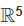Solution. Clearly if Wc is a linear subspace then u + v ∈ Wc whenever u, v ∈ Wc , so that u + v should also satisfy the given diﬀerential equation. So, u′ = xu + c, v′ = xv + c and u′ + v′ = x(u + v) + c. Hence, 2c = c ⇒ c = 0.

Question 20. Let U and V both be two-dimensional subspaces ofand U ≠ V , and let W = U ∩ V . Find all possible values for the dimension of W.

Solution.:  Here, dim(U ) = 2 and dim(V ) = 2. Let BU = {e1, e2} and BV = {e3, e4 } be bases of U and V respectively. It may happen that {e1 , e2 , e3, e4 } is LI, in which case dim(U + V ) = 4 ⇒ dim(U ) + dim(V ) − dim(U ∩ V ) = 4 ⇒ dim(U ∩ V ) = 0.

Again, it may happen that {e1 , e2 , e3, e4 } is LD but {e1, e2 , e3 } is LI, in which case dim(U + V ) = 3 ⇒ dim(U ∩ V ) = 1.

Question 21. Let Pn be the vector space of all polynomials of degree at most n ≥ 6 with real coeﬃcients. Consider the following subspaces of Pn

W= {p(x) ∈ Pn : p(1) = 0, p(2) = 0, p(3) = 0, p(4) = 0}

W2 = {p(x) ∈ Pn : p(3) = 0, p(4) = 0, p(5) = 0, p(6) = 0}

Find the dimensions of W1, W2 and W1 ∩ W2

Solution. Here,

W1 = {p(x) ∈ Pn : p(x) = (x − 1)(x − 2)(x − 3)(x − 4)q(x) where q(x) ∈ Pn−4 }

W1 = {p(x) ∈ Pn : p(x) = (x − 3)(x − 4)(x − 5)(x − 6)q(x) where q(x) ∈ Pn−4 }

Thus, dim(W1 ) = dim(W2) = n − 3. Now, W1 ∩ W2 contains all polynomials of the form
p(x) = (x − 1)(x − 2)(x − 3)(x − 4)(x − 5)(x − 6)q(x), where q(x) ∈ Pn−6
Thus, dim(W∩ W2 ) = n − 5

Question 22. Let P3 denote the real vector space of all polynomials with real coeﬃcients having degree less than or equal to 3, equipped with the standard ordered basis {1, x, x2 , x3 }. Write down the matrix, w.r.t this basis,of the fol lowing linear transformation:

L(p) = p′′ − 2p′ + p, p ∈ P3

Also, ﬁnd the unique polynomial p such that L(p) = x3.

Solution. Here,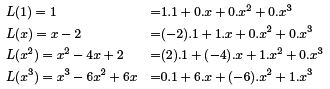Thus matrix of L =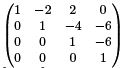Now let p = ax+ bx2 + cx + d ∈ Pbe such the L(p) = x3 . Then, using the deﬁnition of L and equating the coeﬃcients on both sides, we get p = x3 + 6x2 + 18x + 24.

Question 23. A non-zero matrix A ∈ Mn(R) is said to be nilpotent if A= 0 for some positive integer k ≥ 2. If A is nilpotent, which of the fol lowing statements are true? 1. k ≤ n for the smal lest such k. 2. The matrix I + A is invertible. 3. Al l the eigenvalues of A are zero.

Solution : Clearly, Ak has all its eigenvalues equal to zero. But eigenvalues of Ak are just the kth power of the eigenvalues of A. Thus, all the eigenvalues of A must be zero. Hence (3) is true. The eigenvalues of I + A are 1 + 0, 1 + 0, 1 + 0 i.e. 1, 1, 1 and so it is invertible. In fact (I +A)−1 = I −A+A2 −A3 +. . .+(−1)k−1Ak .
Hence, (2) is true. Since all the eigenvalues of A are zero, so the characteristic equation of A is x= 0 and so by Cayley Hamilton theorem, An = 0. Thus, the smallest such k for which Ak = 0 can at most be equal to n. Thus, k ≤ n.
Hence, (1) is also true.

Question 24. Justify whether the fol lowing are true or false: 1. There exist n × n matrices A and B such that AB − BA = I .

2. Let A and B be two arbitrary n × n matrices. If B is invertible, then tr(A) = tr−1 (B − 1AB) where tr(M ) denotes the trace of an n × n matrix M.

Solution. (1) is false. Suppose there exist such matrices. Then tr(AB − B A) = tr(I ) ⇒ tr(AB) − tr(BA) = n. But tr(AB) = tr(BA) and so we would end up with 0 = n which is absurd. Hence there cannot exist such matrices. (2) is true. This is because similar matrices have same eigenvalues and here A and B−1 AB are similar. Thus, tr(A) = sum of the eigenvalues = tr(B−1 AB)

Question 25. Let V be the real vector space of all polynomials in one variable with real coeﬃcients and of degree less than, or equal to 5. Let W be the subspace deﬁned by W = {p ∈ V |p(1) = p′(2) = 0}. What is the dimension of W ?
Solution.
Since p(1) = 0, so (x − 1) is a factor of p(x) ∈ V . thus, p(x) = (x − 1)q(x) where deg(q(x)) ≤ 4. Now, p′(2) = 0 implies that q(2) + q′(2) = 0.
Now, q(x) = (x − 2)g(x) + q(2) where deg(g(x)) ≤ 3.
Thus, the elements in W are of the form p(x) = (x − 1)(x − 2)g(x) + q(2)(x − 1).
But since W is a subspace, so we must have q(2) = 0. Thus, q′(2) = 0 and any element of W is of the form p(x) = (x − 1)(x − 2)g(x), where g(x) is a polynomial of degree at most 3. So, dimension of W is 4.

The document Questions:Linear Algebra and Matrices - Mathematical Methods of Physics, UGC - NET Physics Physics Notes | EduRev is a part of the Physics Course Physics for IIT JAM, UGC - NET, CSIR NET.
All you need of Physics at this link: PhysicsUse Code STAYHOME200 and get INR 200 additional OFF Use Coupon Code
All Tests, Videos & Notes of Physics: Physics## Physics for IIT JAM, UGC - NET, CSIR NET

159 docs

Track your progress, build streaks, highlight & save important lessons and more!

,

,

,

,

,

,

,

,

,

,

,

,

,

,

,

,

,

,

,

,

,

,

,

,

;